# Simple harmonic motion Questions and Answers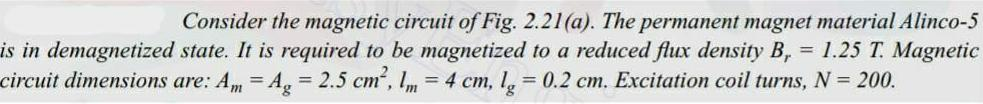Physics
Simple harmonic motion
Consider the magnetic circuit of Fig 2 21 a The permanent magnet material Alinco 5 is in demagnetized state It is required to be magnetized to a reduced flux density B 1 25 T Magnetic circuit dimensions are Am Ag 2 5 cm 1m 4 cm lg 0 2 cm Excitation coil turns N 200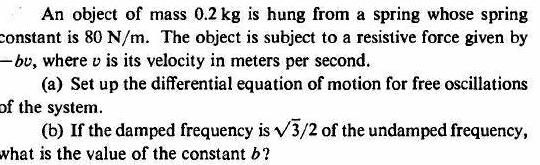Physics
Simple harmonic motion
An object of mass 0 2 kg is hung from a spring whose spring constant is 80 N m The object is subject to a resistive force given by bu where v is its velocity in meters per second a Set up the differential equation of motion for free oscillations of the system b If the damped frequency is 3 2 of the undamped frequency what is the value of the constant b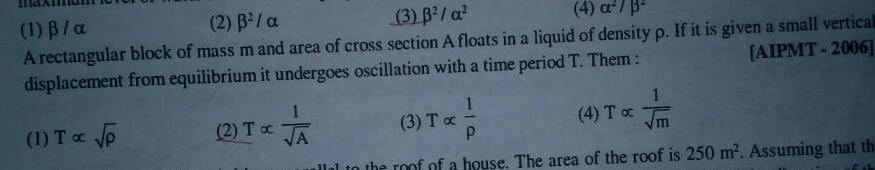Physics
Simple harmonic motion
1 B a 2 3 a 3 B a 4 a 3 A rectangular block of mass m and area of cross section A floats in a liquid of density p If it is given a small verticall displacement from equilibrium it undergoes oscillation with a time period T Them AIPMT 2006 1 T p 2 To A 1 3 Tx 4 To P allal to the roof of a house The area of the roof is 250 m Assuming that th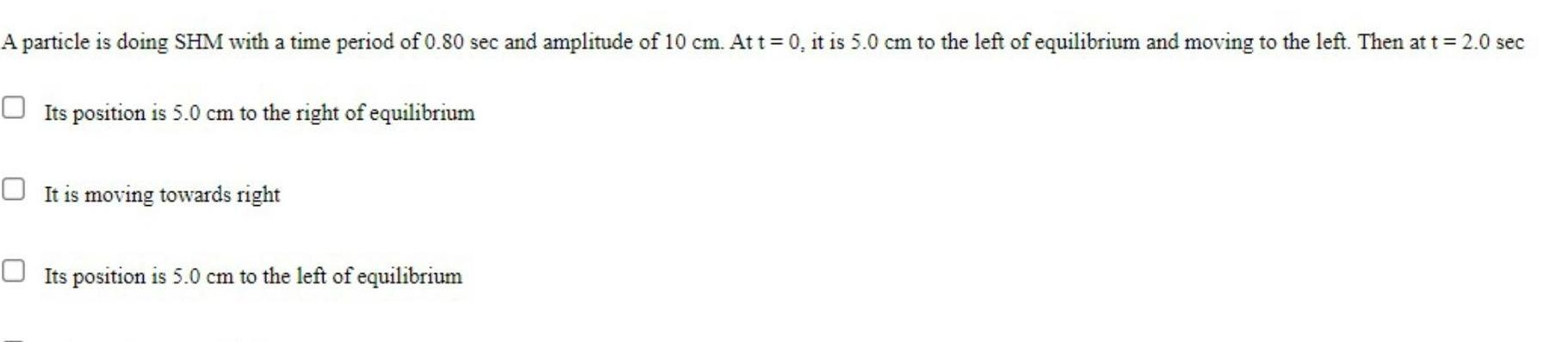Physics
Simple harmonic motion
A particle is doing SHM with a time period of 0 80 sec and amplitude of 10 cm At t 0 it is 5 0 cm to the left of equilibrium and moving to the left Then at t 2 0 sec Its position is 5 0 cm to the right of equilibrium It is moving towards right Its position is 5 0 cm to the left of equilibrium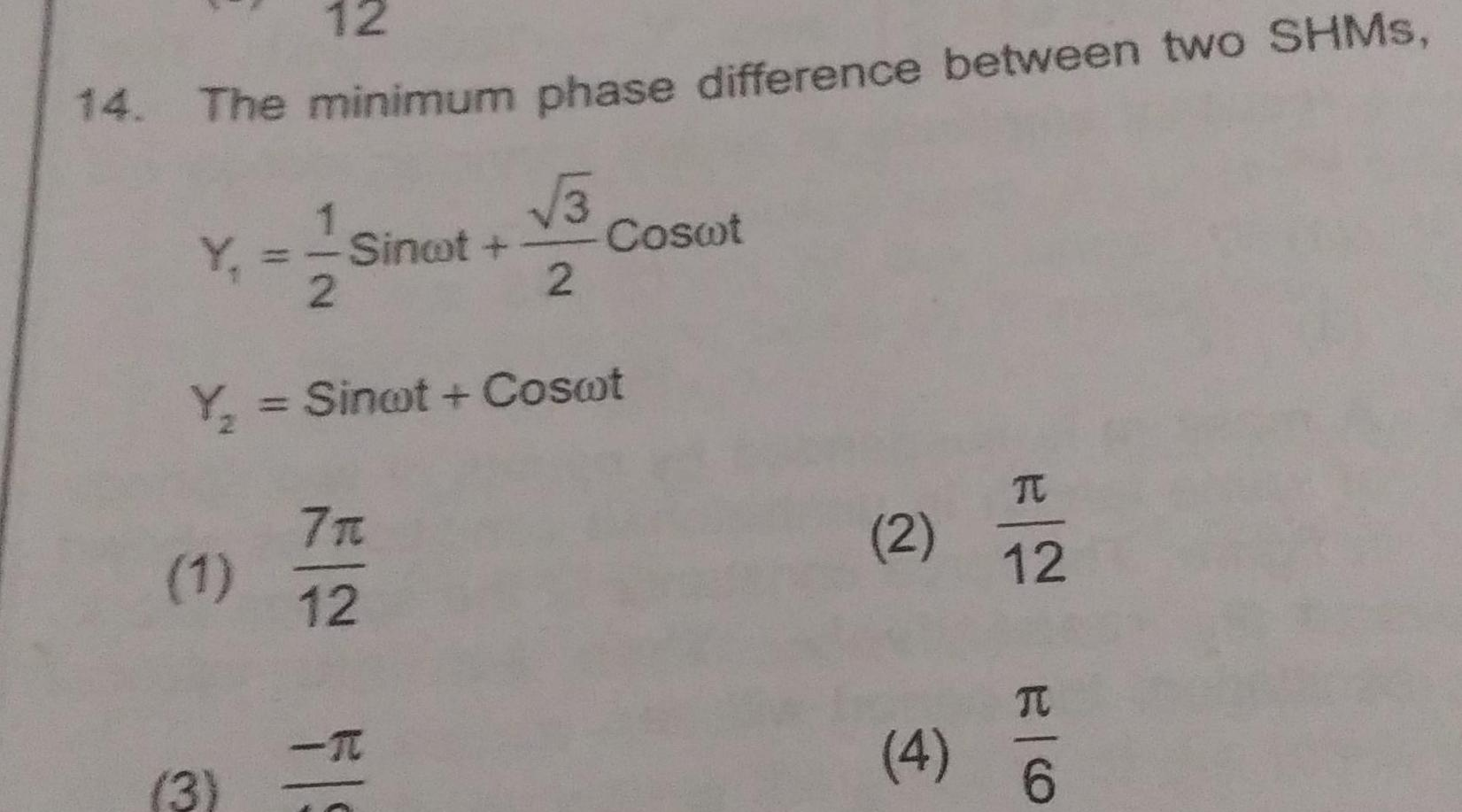Physics
Simple harmonic motion
12 14 The minimum phase difference between two SHMS 1 Y Sinot 2 1 Y Sinot Coswt 3 E FS 7 3 2 12 Coswt 2 4 TC 12 E O TU 6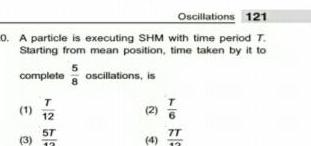Physics
Simple harmonic motion
Oscillations 121 0 A particle is executing SHM with time period T Starting from mean position time taken by it to 5 complete oscillations is 1 3 T 12 5T 12 2 4 7T 12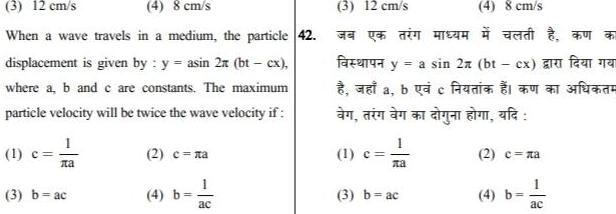Physics
Simple harmonic motion
3 12 cm s 4 8 cm s When a wave travels in a medium the particle 42 displacement is given by y asin 2x bt cx where a b and c are constants The maximum particle velocity will be twice the wave velocity if 1 c 3 b ac 2 a 4 b ac 3 12 cm s 2 y a sin 2x bt cx a fe a bac C n din n an yu m afa fazen Me 1 c 4 8 cm s 3 b ac 2 na 4 b ac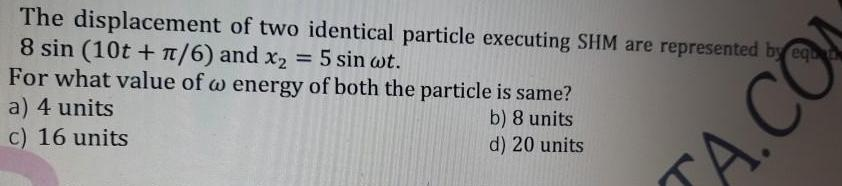Physics
Simple harmonic motion
The displacement of two identical particle executing SHM are represented by equ 8 sin 10t 6 and x 5 sin wt For what value of w energy of both the particle is same a 4 units b 8 units d 20 units c 16 units A CO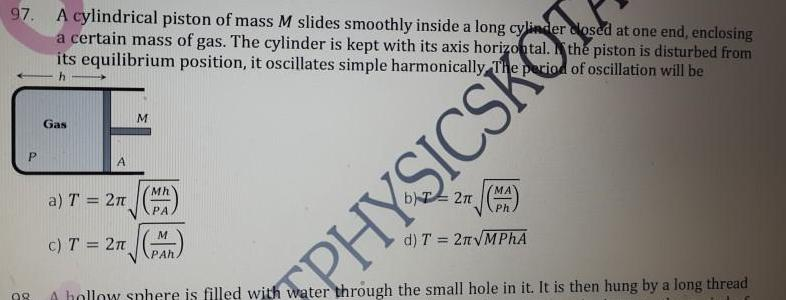Physics
Simple harmonic motion
97 A cylindrical piston of mass M slides smoothly inside a long cylinder a certain mass of gas The cylinder is kept with its axis horizontal closed at one end enclosing the piston is disturbed from its equilibrium position it oscillates simple harmonically The period of oscillation will be h P 98 Gas A a T 2 c T 2 M Mh PA M PAh PHYSICSKOT 2 MA Ph d T 2n MPhA A hollow sphere is filled with water through the small hole in it It is then hung by a long thread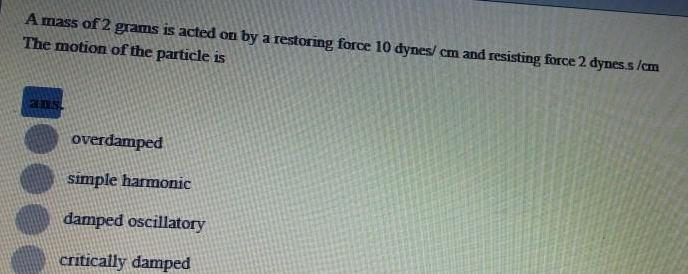Physics
Simple harmonic motion
A mass of 2 grams is acted on by a restoring force 10 dynes cm and resisting force 2 dynes s cm The motion of the particle is 205 overdamped simple harmonic damped oscillatory critically damped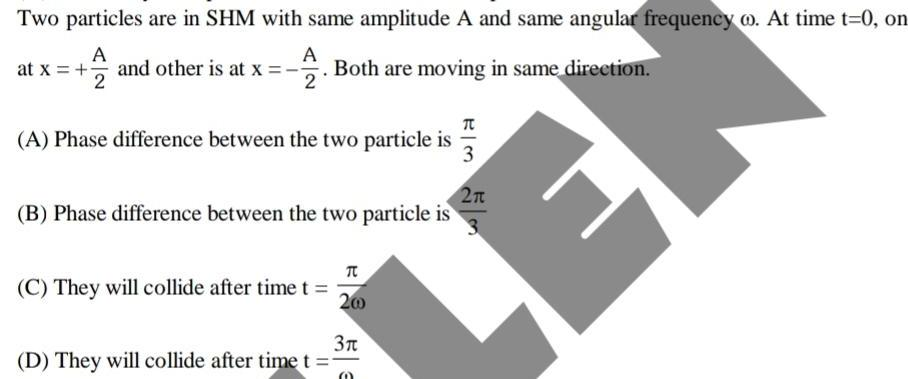Physics
Simple harmonic motion
Two particles are in SHM with same amplitude A and same angular frequency o At time t 0 on A A at x and other is at x Both are moving in same direction 2 2 A Phase difference between the two particle is B Phase difference between the two particle is C They will collide after time t D They will collide after time t NET 200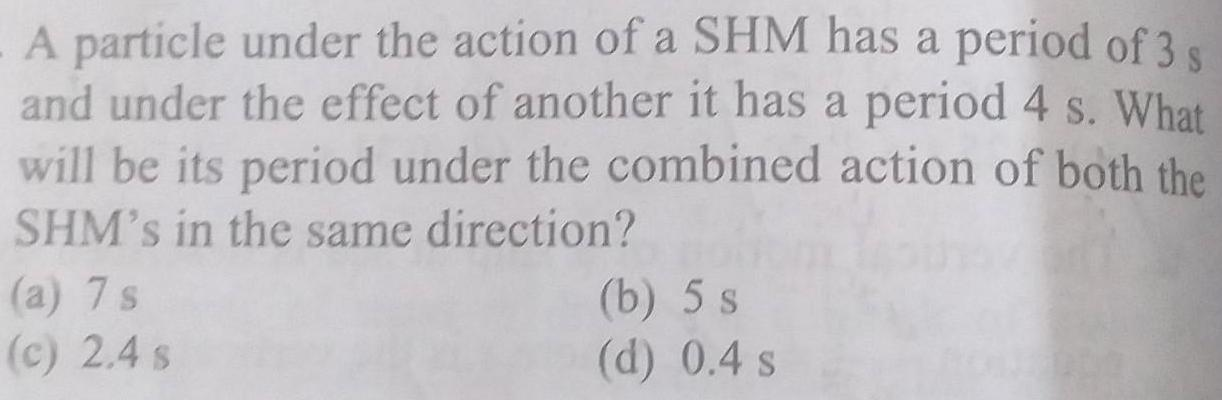Physics
Simple harmonic motion
A particle under the action of a SHM has a period of 3 s and under the effect of another it has a period 4 s What will be its period under the combined action of both the SHM s in the same direction a 7 s c 2 4 s b 5 s d 0 4 s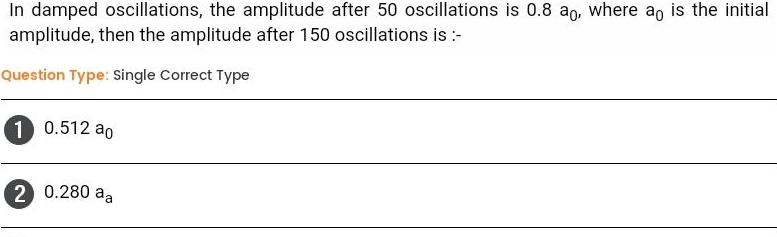Physics
Simple harmonic motion
In damped oscillations the amplitude after 50 oscillations is 0 8 ao where ao is the initial amplitude then the amplitude after 150 oscillations is Question Type Single Correct Type 1 0 512 ao 2 0 280 aa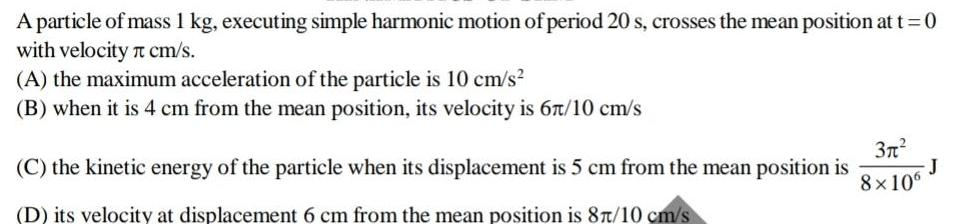Physics
Simple harmonic motion
A particle of mass 1 kg executing simple harmonic motion of period 20 s crosses the mean position at t 0 with velocity cm s A the maximum acceleration of the particle is 10 cm s B when it is 4 cm from the mean position its velocity is 67 10 cm s C the kinetic energy of the particle when its displacement is 5 cm from the mean position is D its velocity at displacement 6 cm from the mean position is 87 10 cm s 3 8x106 J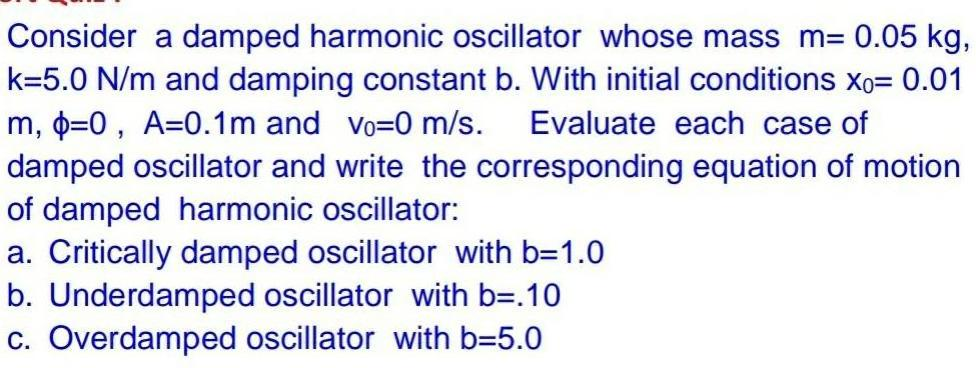Physics
Simple harmonic motion
Consider a damped harmonic oscillator whose mass m 0 05 kg k 5 0 N m and damping constant b With initial conditions xo 0 01 m p 0 A 0 1m and vo 0 m s Evaluate each case of damped oscillator and write the corresponding equation of motion of damped harmonic oscillator a Critically damped oscillator with b 1 0 b Underdamped oscillator with b 10 c Overdamped oscillator with b 5 0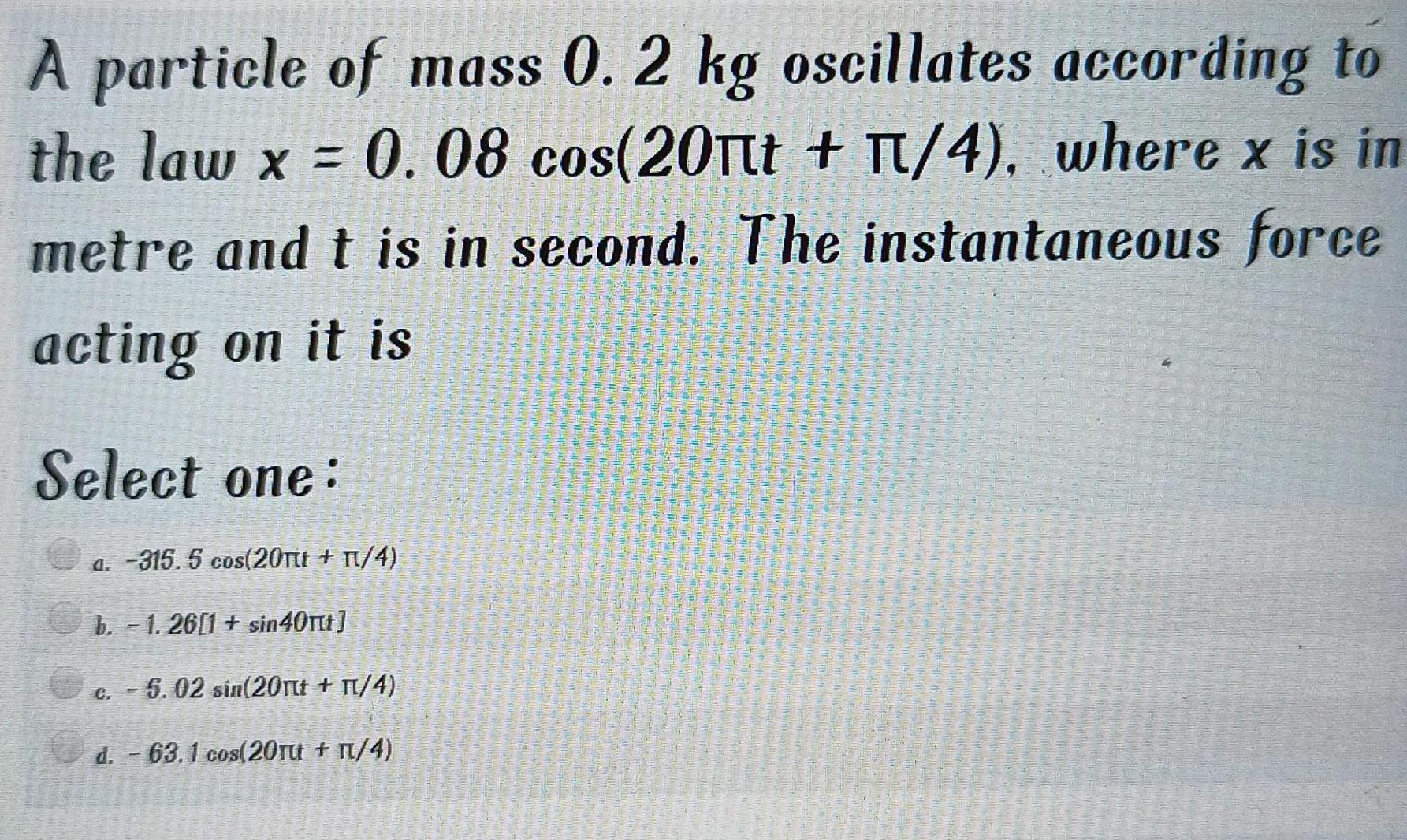Physics
Simple harmonic motion
A particle of mass 0 2 kg oscillates according to the law x 0 08 cos 20 t T 4 where x is in metre and t is in second The instantaneous force acting on it is Select one a 315 5 cos 20nt n 4 b 1 26 1 sin40 t c 5 02 sin 20nt 4 d 63 1 cos 20ru TL 4 LEGE b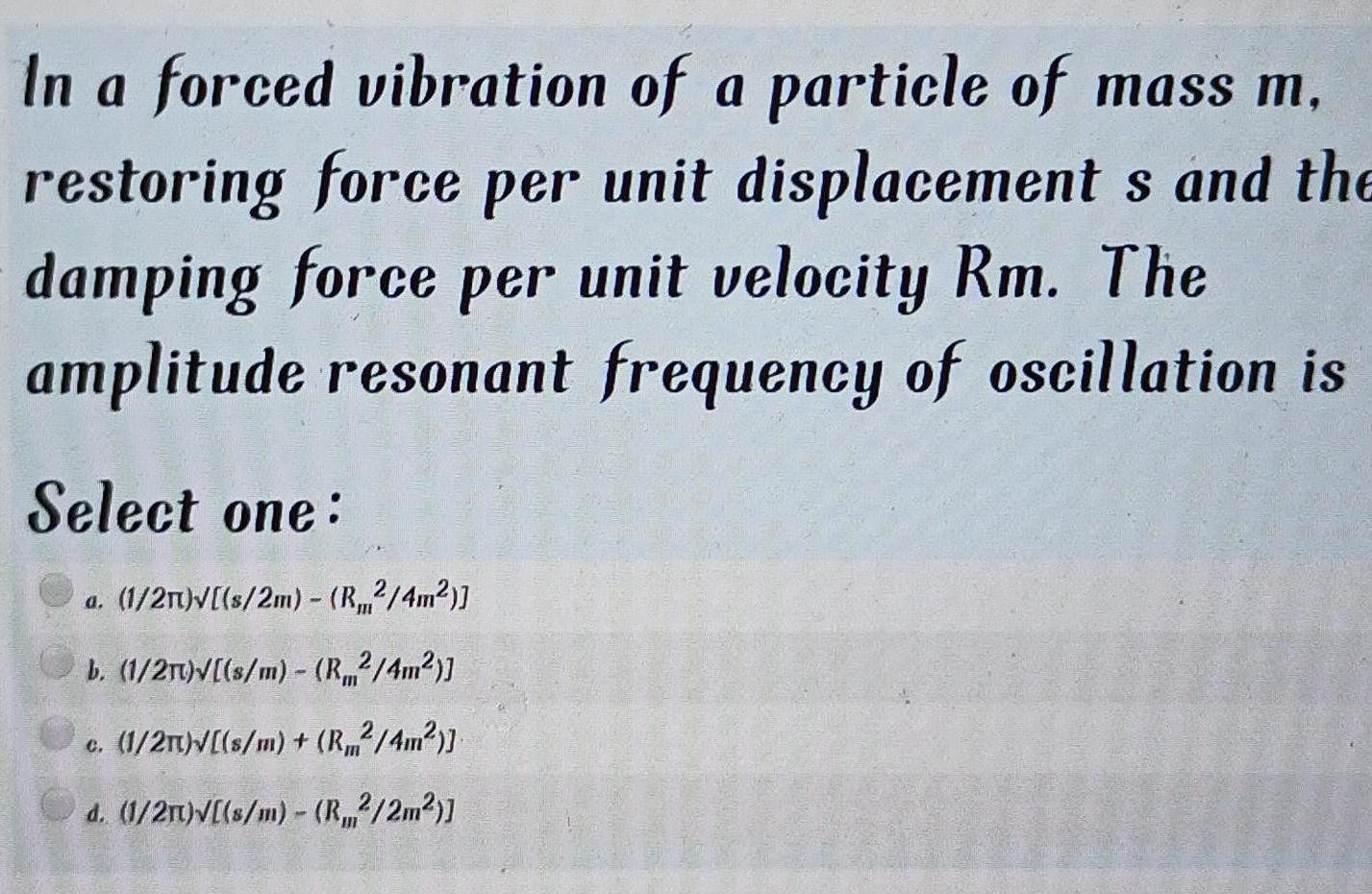Physics
Simple harmonic motion
In a forced vibration of a particle of mass m restoring force per unit displacement s and the damping force per unit velocity Rm The amplitude resonant frequency of oscillation is Select one a 1 21 s 2m R2 4m b 1 2T s m R 4m c 1 2 s m R 4m d 1 2m s m R2 2m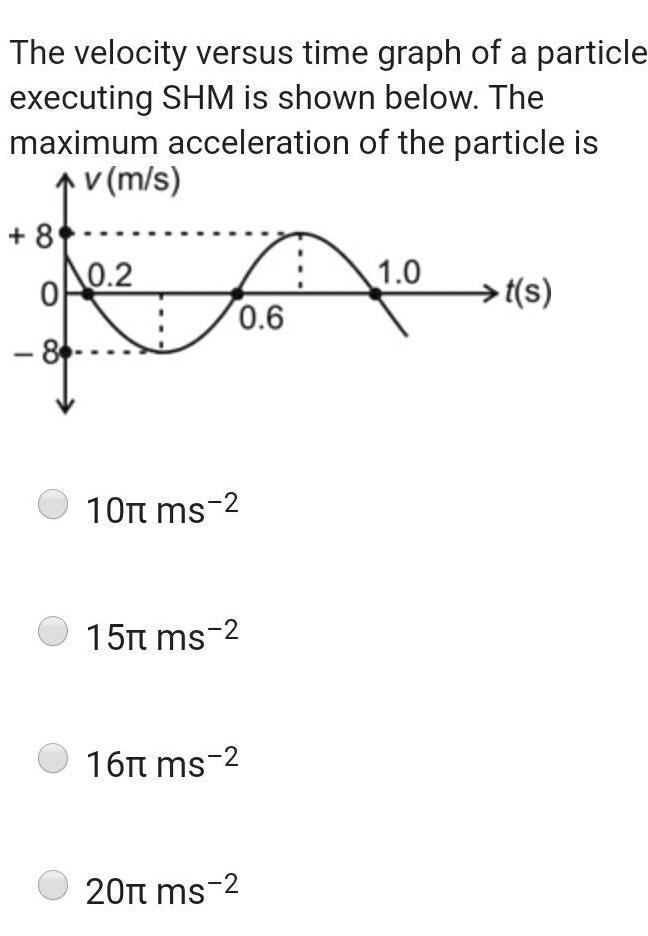Physics
Simple harmonic motion
The velocity versus time graph of a particle executing SHM is shown below The maximum acceleration of the particle is v m s 8 0 86 0 2 7 foo 0 6 10 ms 2 15 ms 2 16 ms 2 20 ms 2 1 0 t s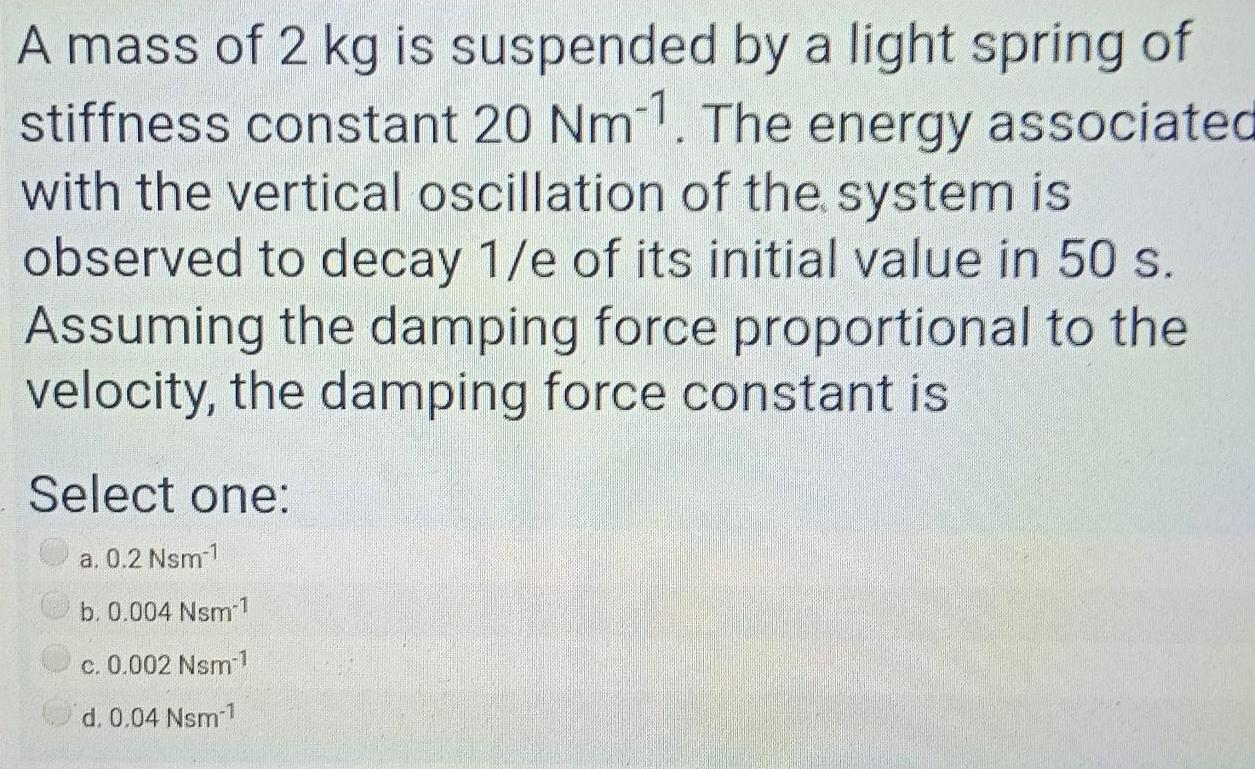Physics
Simple harmonic motion
A mass of 2 kg is suspended by a light spring of stiffness constant 20 Nm11 The energy associated with the vertical oscillation of the system is observed to decay 1 e of its initial value in 50 s Assuming the damping force proportional to the velocity the damping force constant is Select one a 0 2 Nsm 1 b 0 004 Nsm c 0 002 Nsm 1 d 0 04 Nsm 1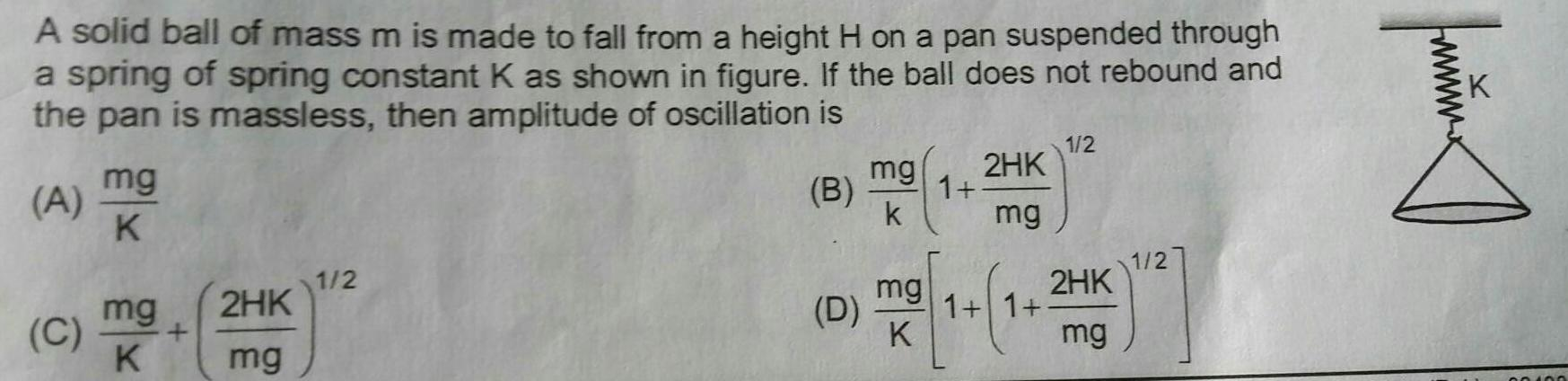Physics
Simple harmonic motion
A solid ball of mass m is made to fall from a height H on a pan suspended through a spring of spring constant K as shown in figure If the ball does not rebound and the pan is massless then amplitude of oscillation is ABA A mg K mg C K 2HK mg 1 2 B D mg k mg K 1 2HK mg 1 1 1 2 2HK mg 1 2 fmmx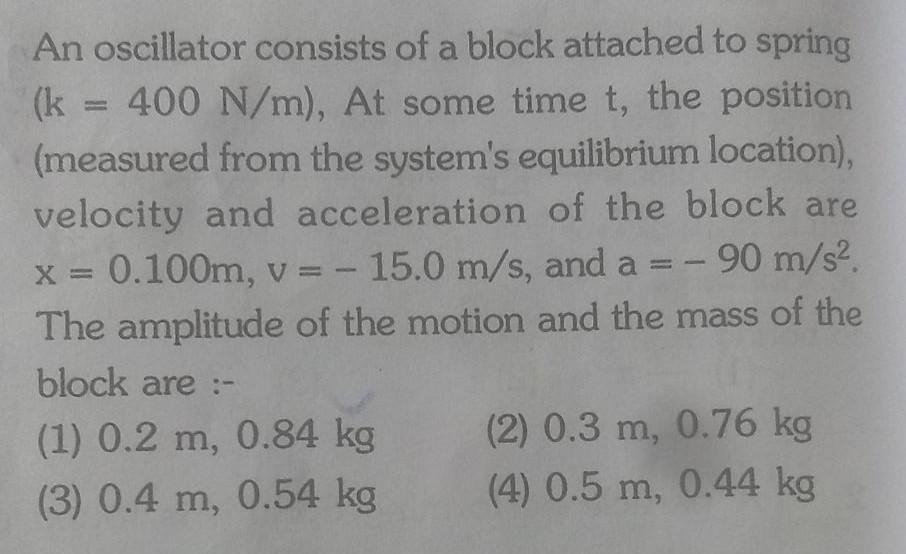Physics
Simple harmonic motion
An oscillator consists of a block attached to spring k 400 N m At some time t the position measured from the system s equilibrium location velocity and acceleration of the block are x 0 100m v 15 0 m s and a 90 m s The amplitude of the motion and the mass of the block are 1 0 2 m 0 84 kg 3 0 4 m 0 54 kg 2 0 3 m 0 76 kg 4 0 5 m 0 44 kg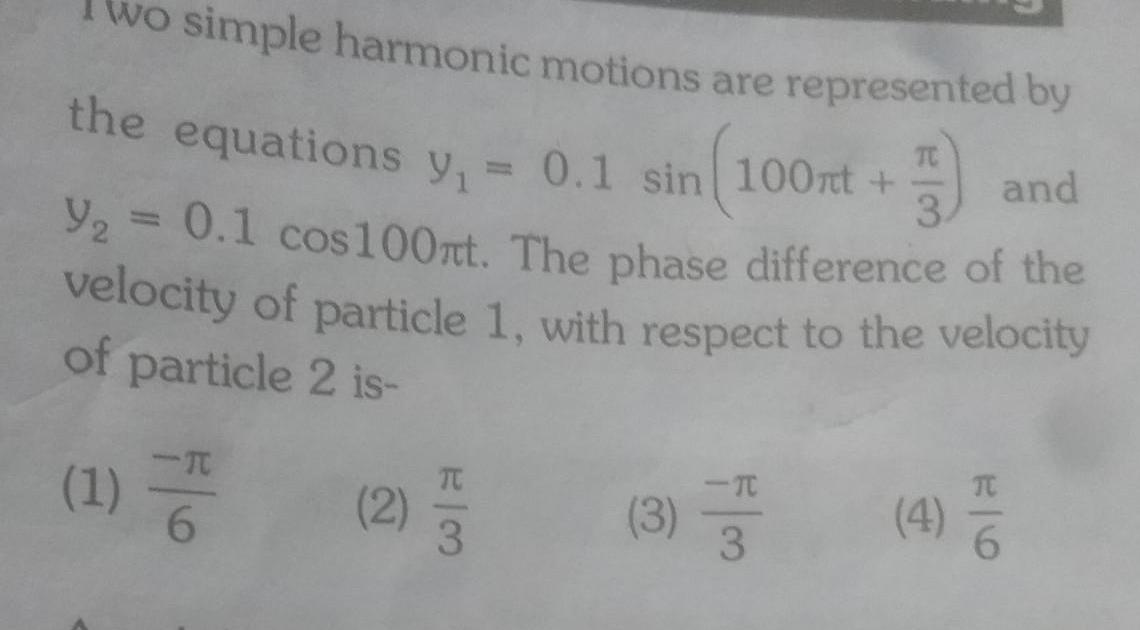Physics
Simple harmonic motion
wo simple harmonic motions are represented by the equations y 0 1 sin 100nt F 3 and Y 0 1 cos100nt The phase difference of the velocity of particle 1 with respect to the velocity of particle 2 is 1 2 3 3 3 4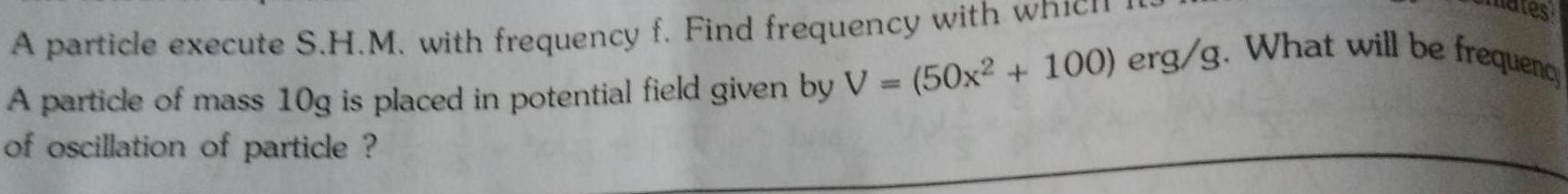Physics
Simple harmonic motion
res A particle execute S H M with frequency f Find frequency with A particle of mass 10g is placed in potential field given by V 50x2 100 erg g What will be frequenc of oscillation of particle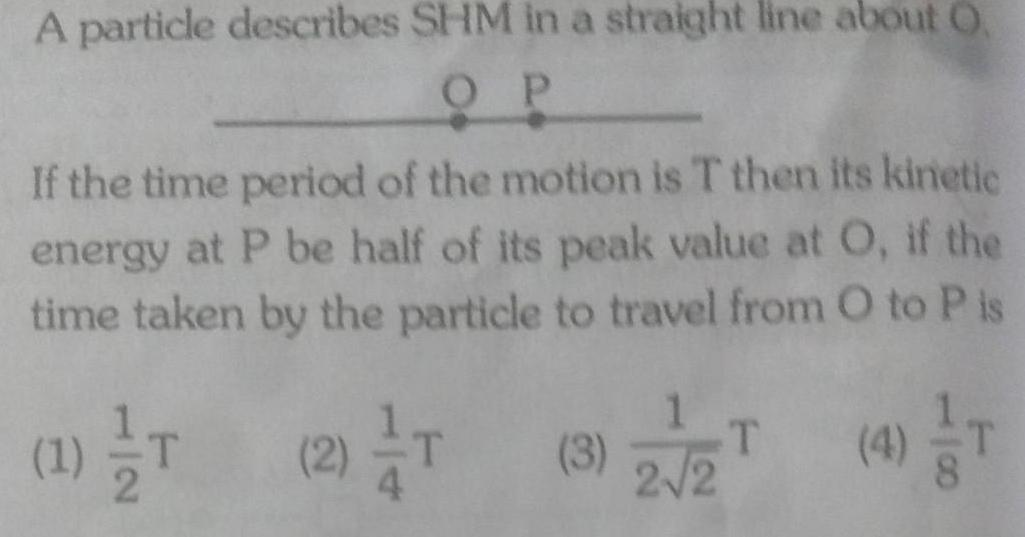Physics
Simple harmonic motion
A particle describes SHM in a straight line about O OP If the time period of the motion is T then its kinetic energy at P be half of its peak value at O if the time taken by the particle to travel from O to P is 4 T 12 1 T T 2 T 3 2 2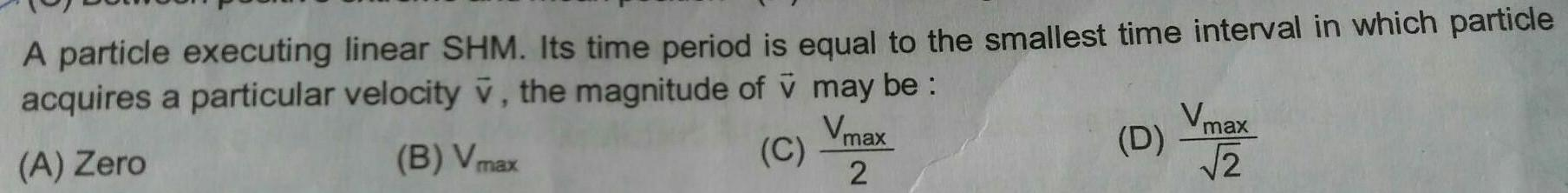Physics
Simple harmonic motion
A particle executing linear SHM Its time period is equal to the smallest time interval in which particle acquires a particular velocity v the magnitude of v may be Vmax B Vmax C A Zero 2 D Vmax 2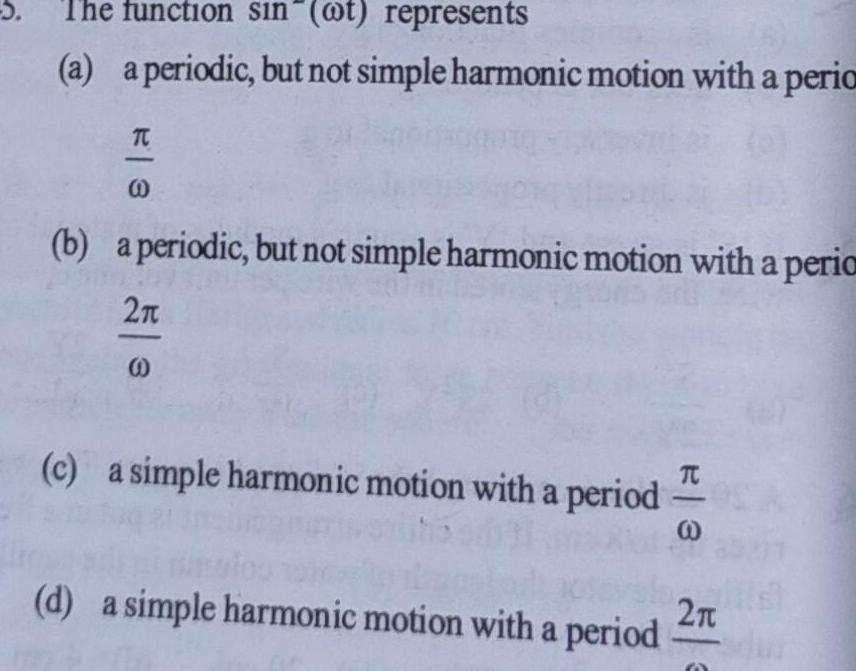Physics
Simple harmonic motion
5 The function sin t represents a a periodic but not simple harmonic motion with a peria TC 0 b a periodic but not simple harmonic motion with a peria 2 c a simple harmonic motion with a period d a simple harmonic motion with a period 3 2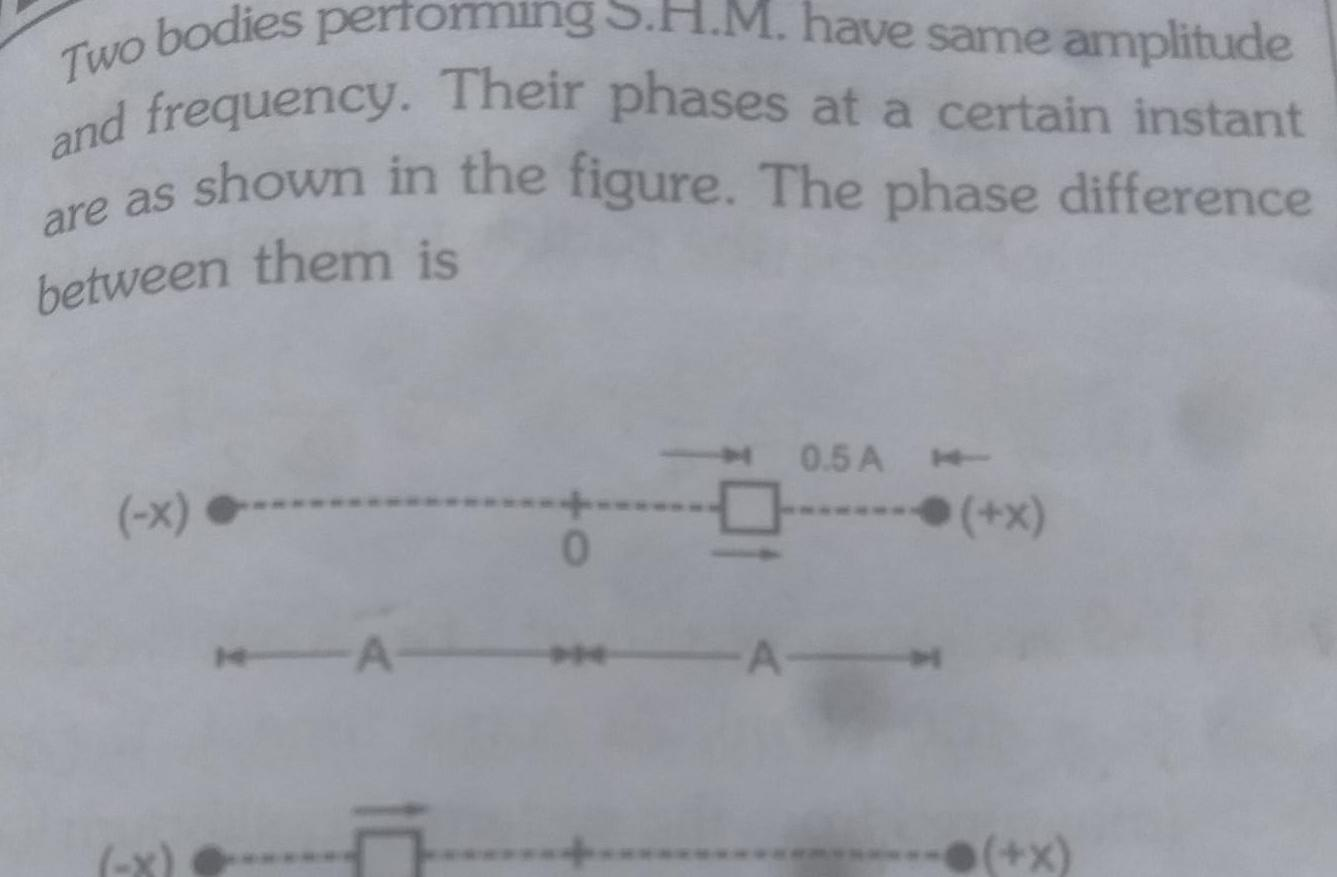Physics
Simple harmonic motion
Two bodies performing have same amplitude and frequency Their phases at a certain instant are as shown in the figure The phase difference between them is x A 10 0 5 A A x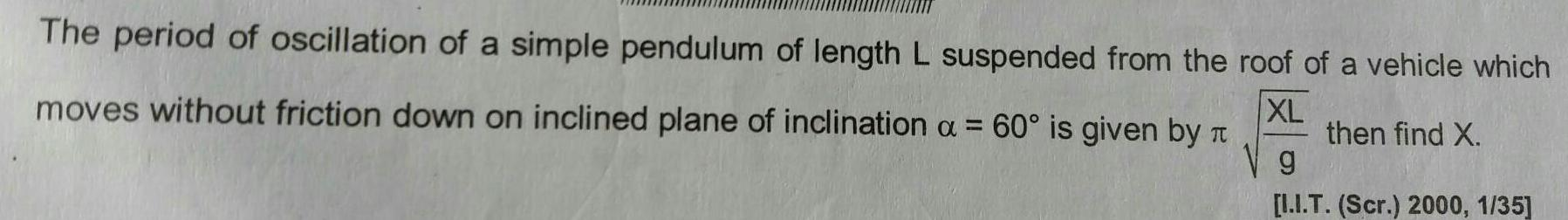Physics
Simple harmonic motion
The period of oscillation of a simple pendulum of length L suspended from the roof of a vehicle which XL then find X moves without friction down on inclined plane of inclination a 60 is given by g I I T Scr 2000 1 35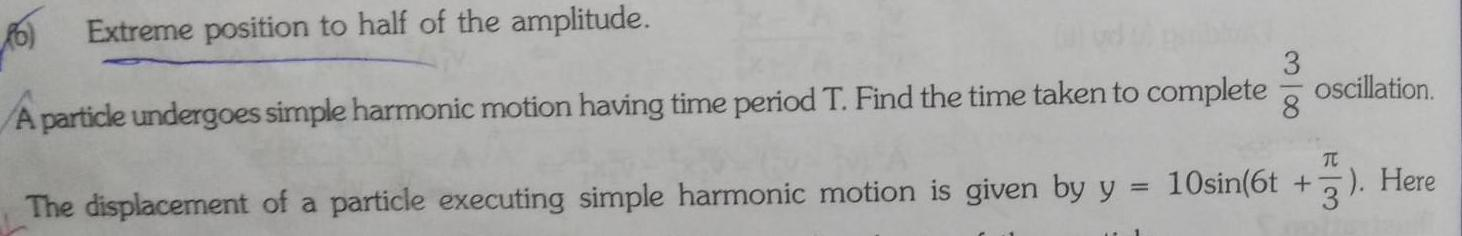Physics
Simple harmonic motion
6 Extreme position to half of the amplitude A particle undergoes simple harmonic motion having time period T Find the time taken to complete 318 oscillation TC The displacement of a particle executing simple harmonic motion is given by y 10sin 6t 3 Here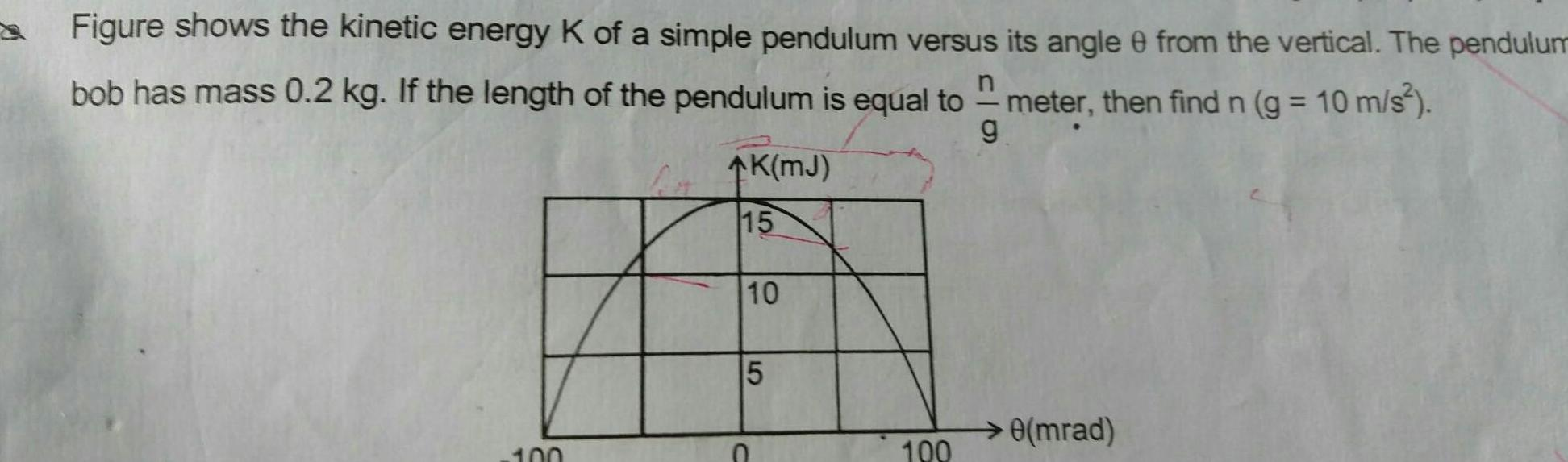Physics
Simple harmonic motion
Figure shows the kinetic energy K of a simple pendulum versus its angle 0 from the vertical The pendulum bob has mass 0 2 kg If the length of the pendulum is equal to g meter then find n g 10 m s 100 K MJ 15 10 5 100 0 mrad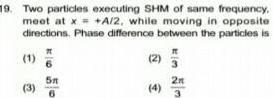Physics
Simple harmonic motion
19 Two particles executing SHM of same frequency meet at x A 2 while moving in opposite directions Phase difference between the particles is It 1 3 51 2 4 2 3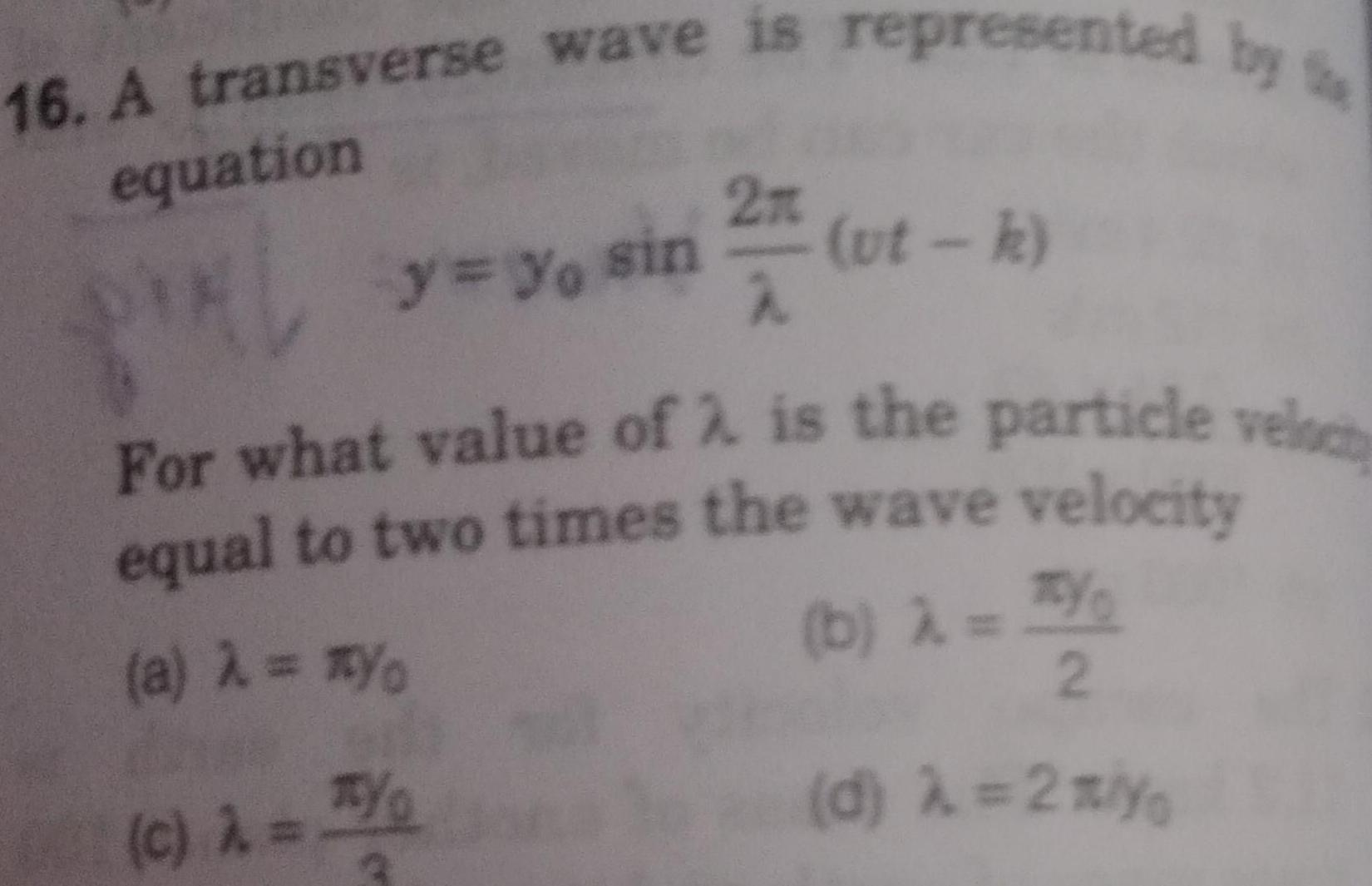Physics
Simple harmonic motion
16 A transverse wave is represented by equation y yo sin 32 74 ut k For what value of 2 is the particle veloc equal to two times the wave velocity a Yo b c TY 2 d 2 yo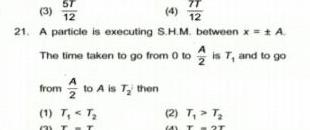Physics
Simple harmonic motion
ST 3 4 12 12 21 A particle is executing S H M between x A The time taken to go from 0 to is T and to go from to A is T then 1 T T T 2 T T T 2T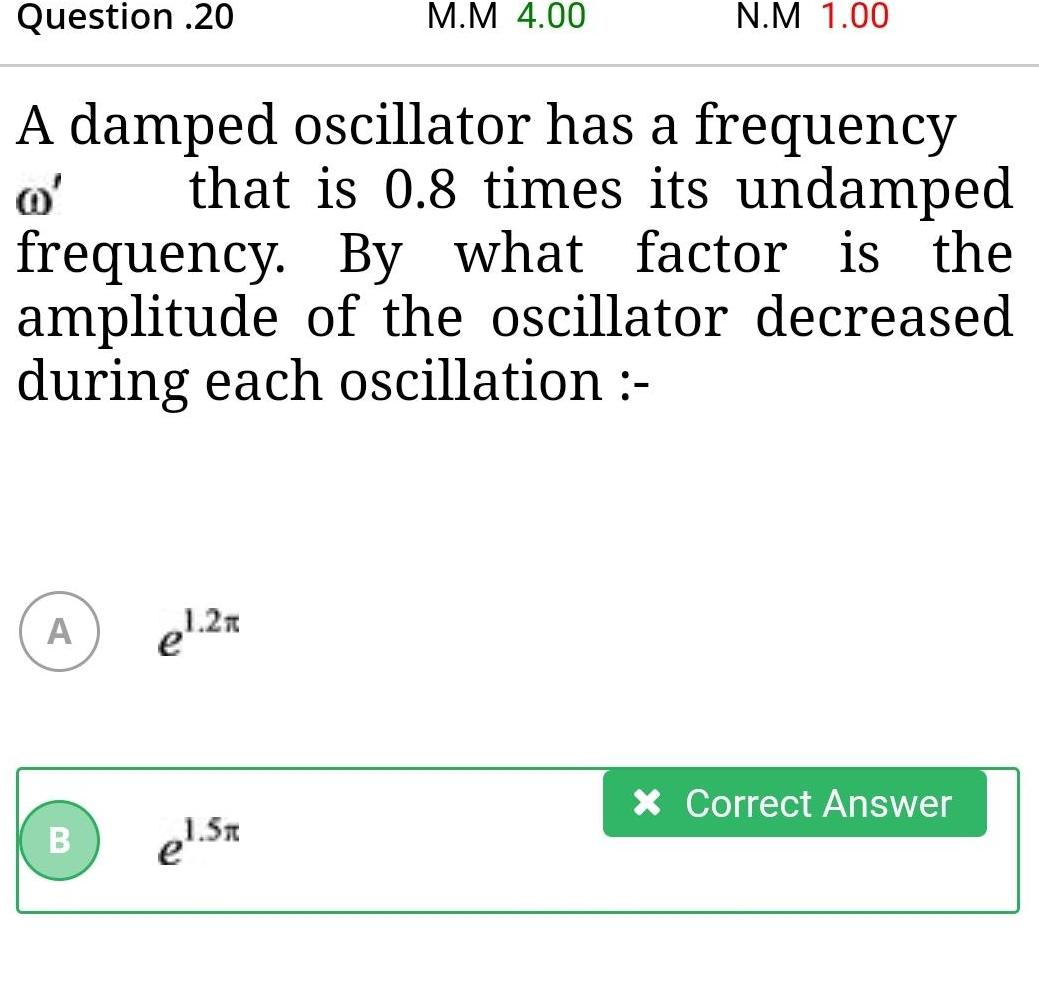Physics
Simple harmonic motion
Question 20 A A damped oscillator has a frequency that is 0 8 times its undamped frequency By what factor is the amplitude of the oscillator decreased during each oscillation B el 2x M M 4 00 1 5x N M 1 00 Correct Answer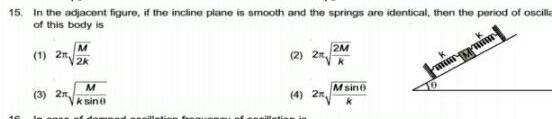Physics
Simple harmonic motion
15 In the adjacent figure if the incline plane is smooth and the springs are identical then the period of oscilla of this body is 1 2n 3 2 M 2k M ksine 2 4 2 2M Msin 10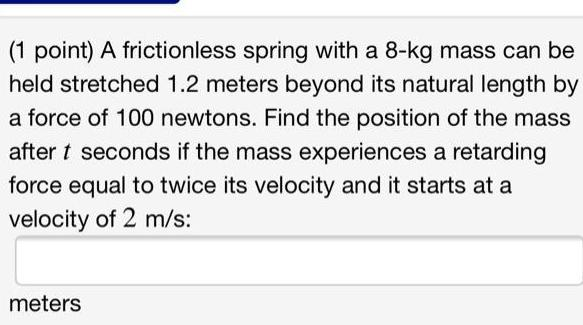Physics
Simple harmonic motion
1 point A frictionless spring with a 8 kg mass can be held stretched 1 2 meters beyond its natural length by a force of 100 newtons Find the position of the mass after t seconds if the mass experiences a retarding force equal to twice its velocity and it starts at a velocity of 2 m s meters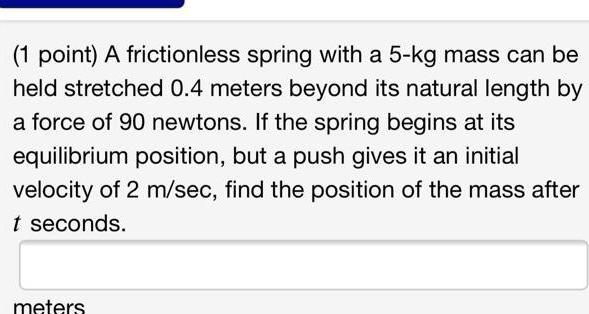Physics
Simple harmonic motion
1 point A frictionless spring with a 5 kg mass can be held stretched 0 4 meters beyond its natural length by a force of 90 newtons If the spring begins at its equilibrium position but a push gives it an initial velocity of 2 m sec find the position of the mass after t seconds meters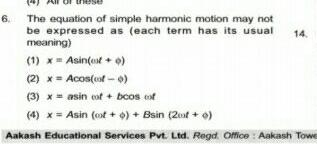Physics
Simple harmonic motion
6 The equation of simple harmonic motion may not be expressed as each term has its usual 14 meaning 1 x Asin et o 2 x Acos oof o 3 xasin cof bcos cof 4 x Asin oot o Bsin 2 0 Aakash Educational Services Pvt Ltd Regd Office Aakash Towe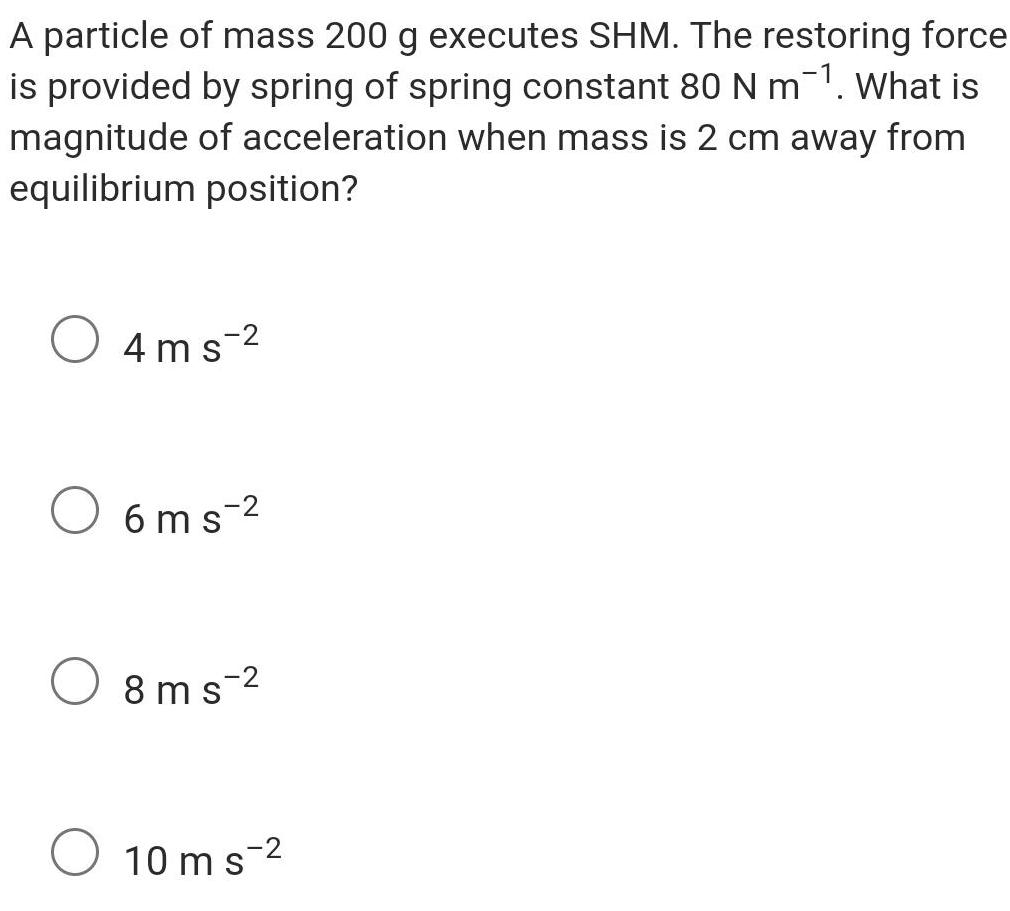Physics
Simple harmonic motion
A particle of mass 200 g executes SHM The restoring force is provided by spring of spring constant 80 N m What is magnitude of acceleration when mass is 2 cm away from equilibrium position 4ms 2 6ms 8ms 2 10 ms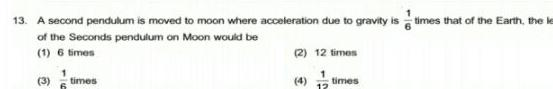Physics
Simple harmonic motion
13 A second pendulum is moved to moon where acceleration due to gravity is times that of the Earth the le of the Seconds pendulum on Moon would be 1 6 times 2 12 times 3 times 12 times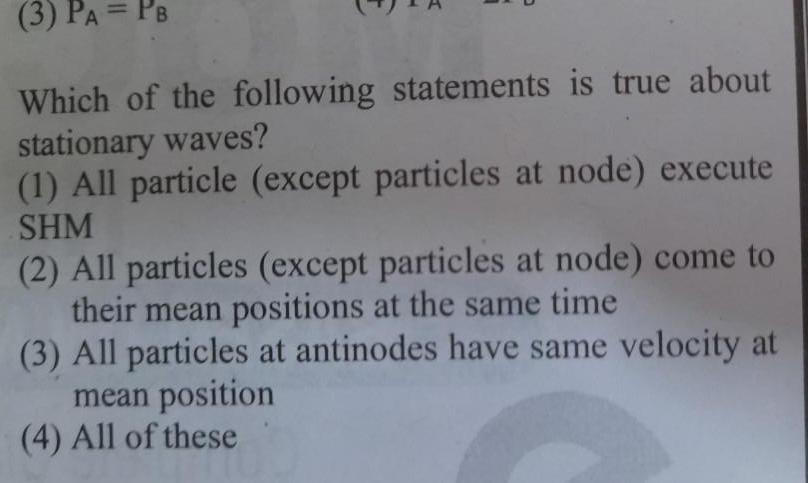Physics
Simple harmonic motion
3 PA PB Which of the following statements is true about stationary waves 1 All particle except particles at node execute SHM 2 All particles except particles at node come to their mean positions at the same time 3 All particles at antinodes have same velocity at mean position 4 All of these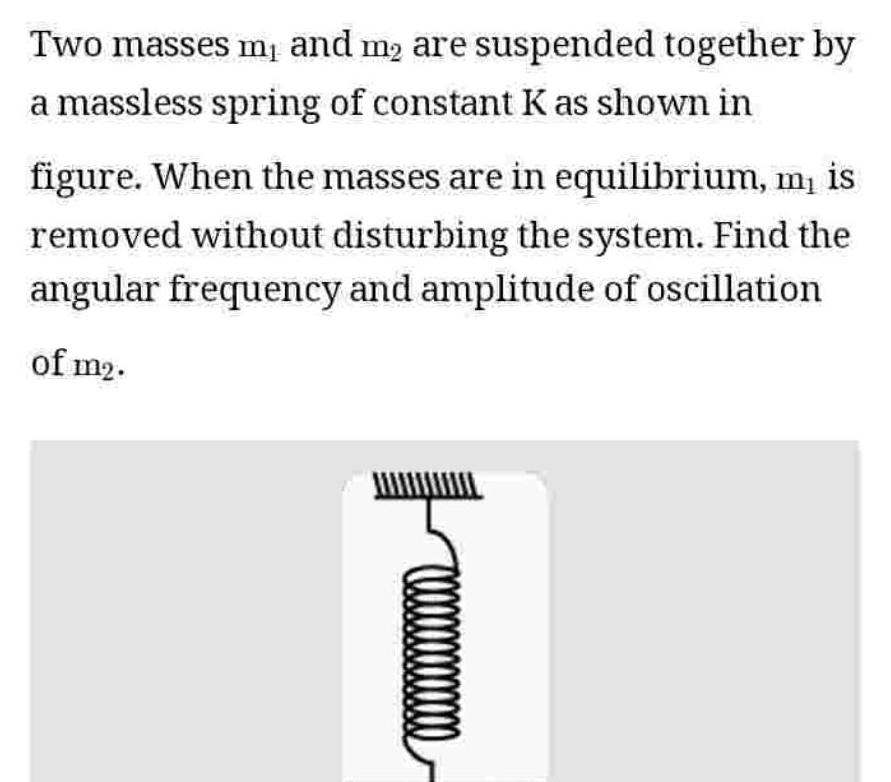Physics
Simple harmonic motion
Two masses m and m are suspended together by a massless spring of constant K as shown in figure When the masses are in equilibrium m is removed without disturbing the system Find the angular frequency and amplitude of oscillation of m2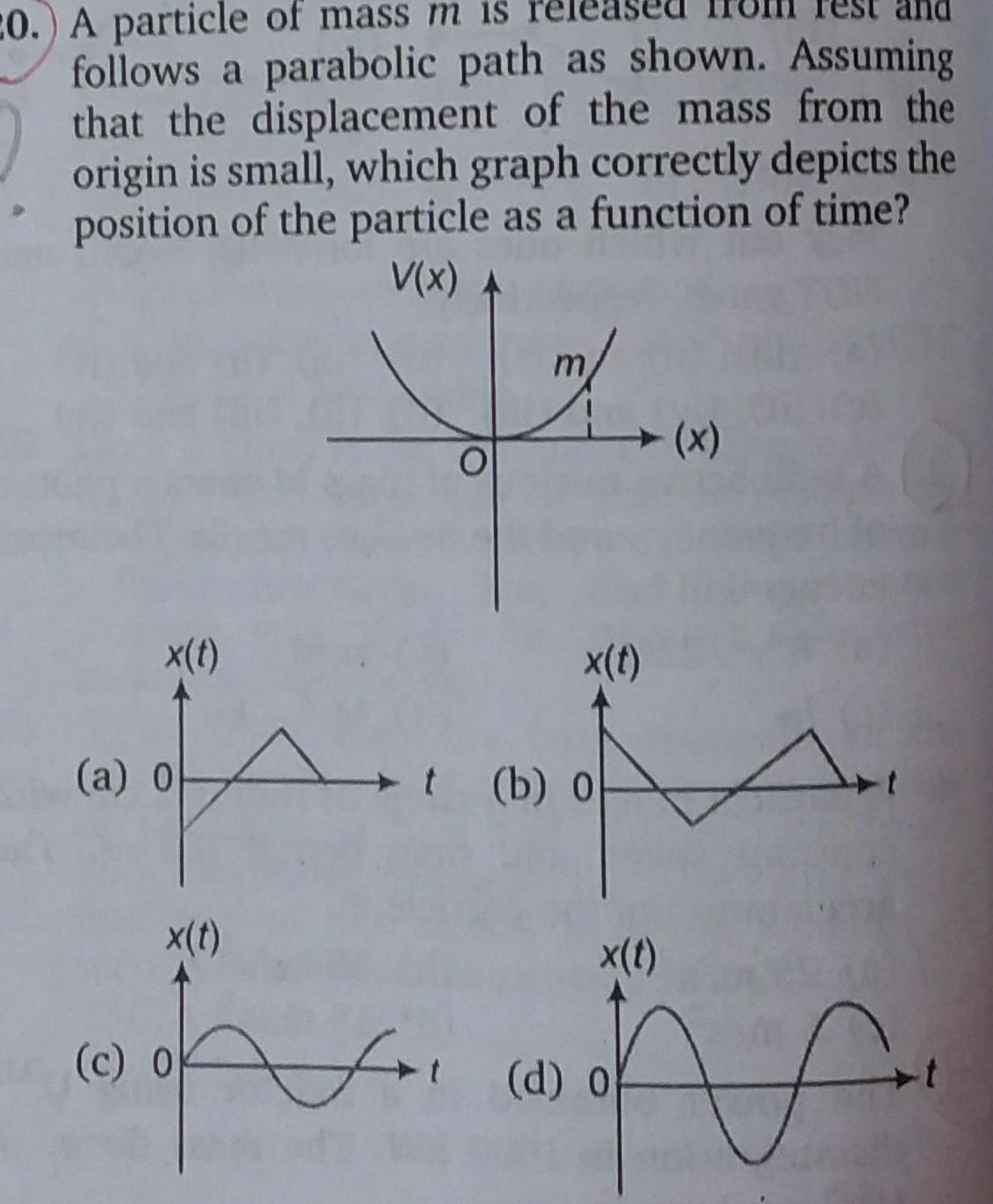Physics
Simple harmonic motion
20 A particle of mass m is released follows a parabolic path as shown Assuming that the displacement of the mass from the origin is small which graph correctly depicts the position of the particle as a function of time V x x t a o x t c 0 O x t b o x x t d o for t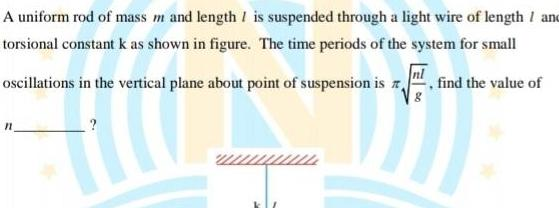Physics
Simple harmonic motion
A uniform rod of mass m and length I is suspended through a light wire of length and torsional constant k as shown in figure The time periods of the system for small oscillations in the vertical plane about point of suspension is find the value of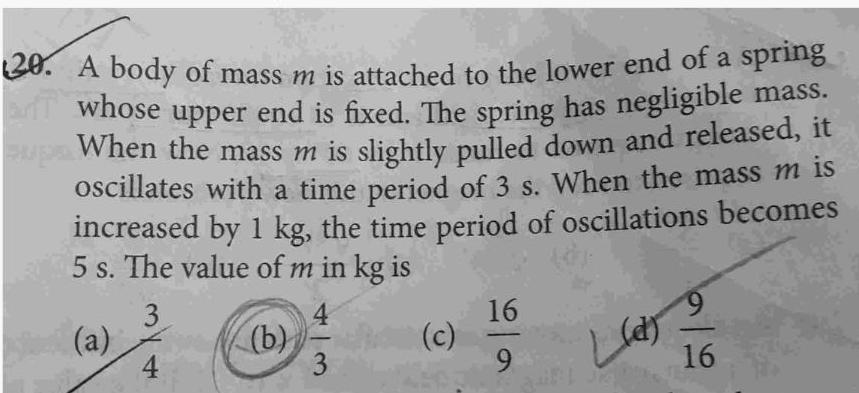Physics
Simple harmonic motion
20 A body of mass m is attached to the lower end of a spring an whose upper end is fixed The spring has negligible mass supe When the mass m is slightly pulled down and released it oscillates with a time period of 3 s When the mass m is increased by 1 kg the time period of oscillations becomes 5 s The value of m in kg is 3 a 4 b 4 3 c 16 9 9 Lun 22 16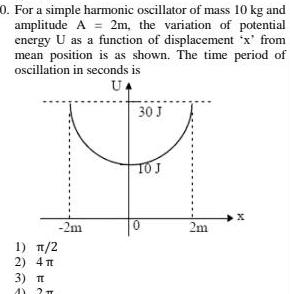Physics
Simple harmonic motion
0 For a simple harmonic oscillator of mass 10 kg and amplitude A 2m the variation of potential energy U as a function of displacement x from mean position is as shown The time period of oscillation in seconds is UA 1 2 2 4 3 T AY 2 T 2m 30 J TO J 0 2m X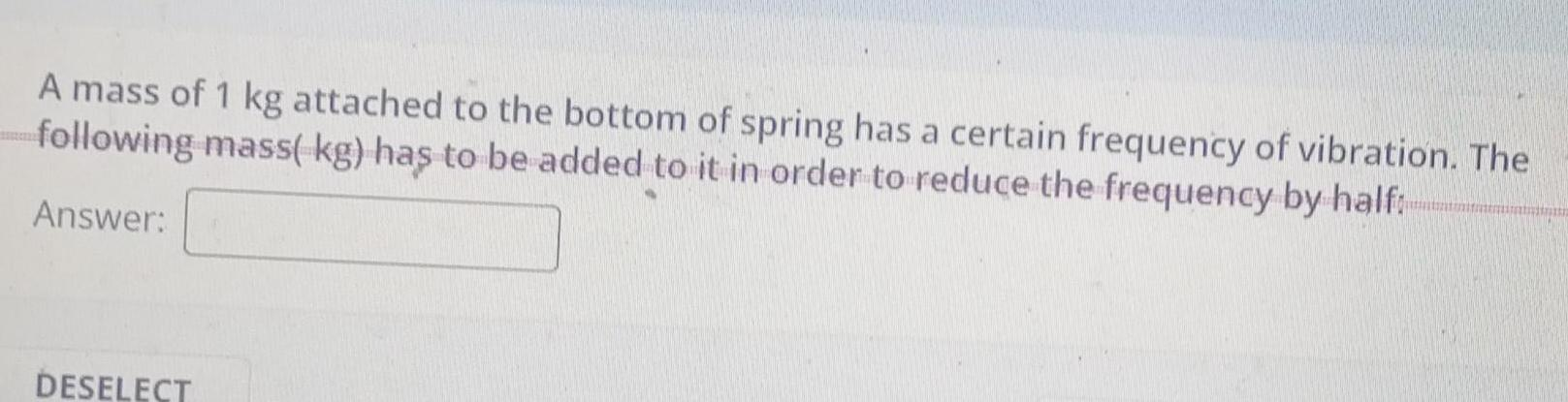Physics
Simple harmonic motion
A mass of 1 kg attached to the bottom of spring has a certain frequency of vibration The following mass kg has to be added to it in order to reduce the frequency by half Answer DESELECT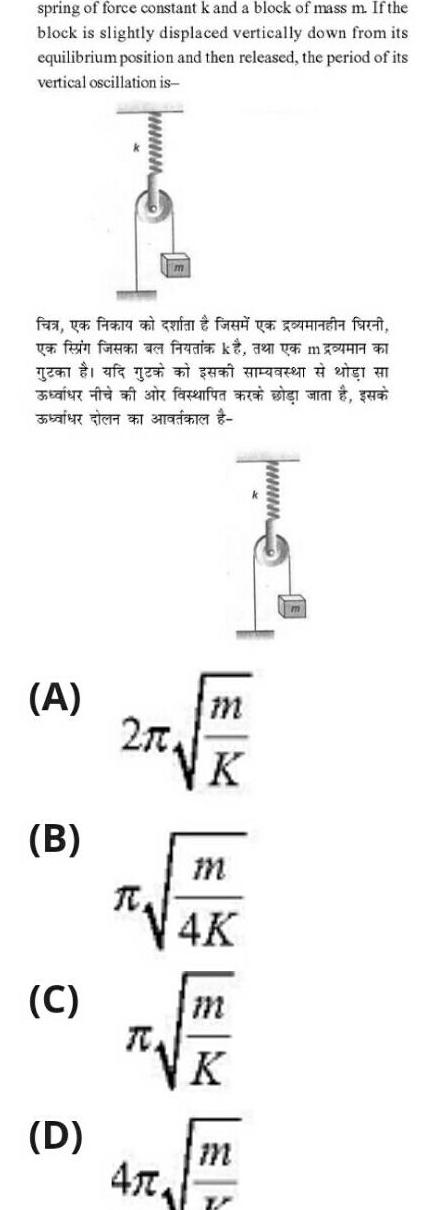Physics
Simple harmonic motion
spring of force constant k and a block of mass m If the block is slightly displaced vertically down from its equilibrium position and then released the period of its vertical oscillation is fea ya fiera an quan fad ya una farci yen fein foren a gen af g a A B C D facenfura a visi m 2 0 K R 47 m 4K K m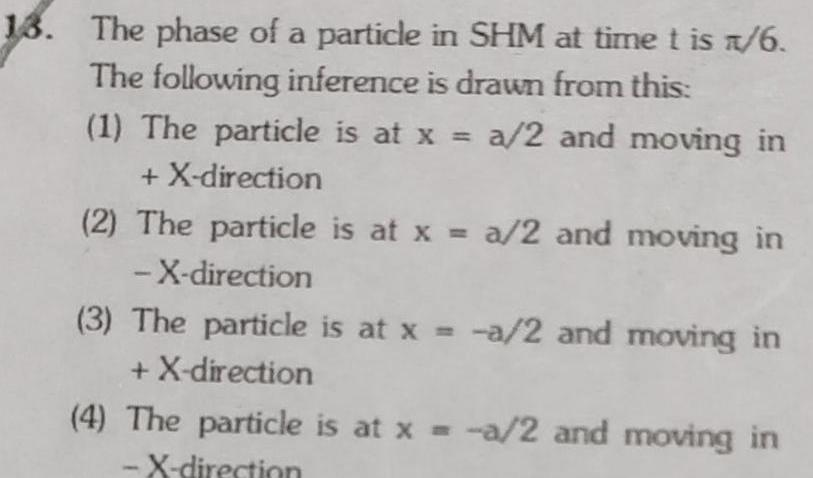Physics
Simple harmonic motion
13 The phase of a particle in SHM at time t is 1 6 The following inference is drawn from this 1 The particle is at x a 2 and moving in X direction 2 The particle is at x a 2 and moving in X direction 3 The particle is at x a 2 and moving in X direction 4 The particle is at x a 2 and moving in X direction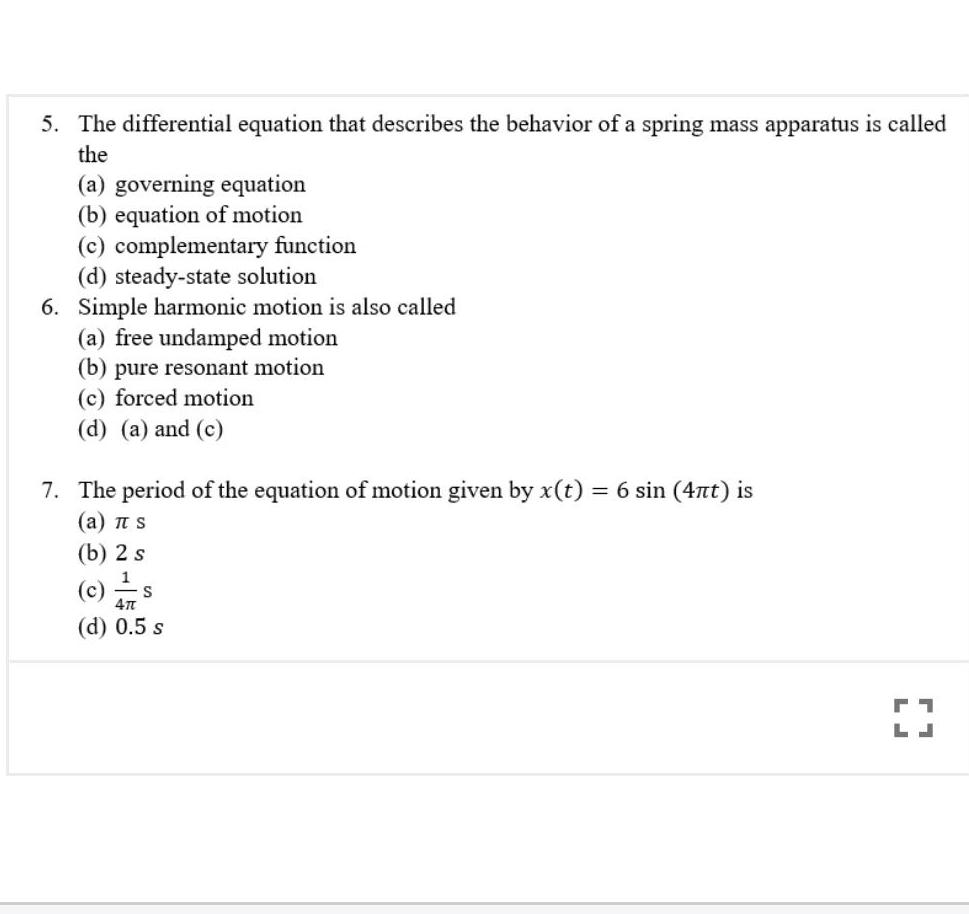Physics
Simple harmonic motion
5 The differential equation that describes the behavior of a spring mass apparatus is called the a governing equation b equation of motion c complementary function d steady state solution 6 Simple harmonic motion is also called a free undamped motion b pure resonant motion c forced motion d a and c 7 The period of the equation of motion given by x t 6 sin 4nt is a TT S b 2 s 1 c 47 d 0 5 s S 71 LJ